Updating search results...

# 37 Results

View
Selected filters:
• Order of Operations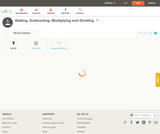Conditional Remix & Share Permitted
CC BY-NC-SA
Rating
0.0 stars

This short video and interactive assessment activity is designed to teach fifth graders about adding, subtracting, multiplying and dividing.

Subject:
Mathematics
Numbers and Operations
Material Type:
Assessment
Interactive
Lecture
Provider:
CK-12 Foundation
Provider Set:
CK-12 Elementary Math
Date Added:
03/06/2015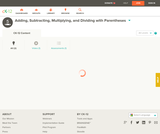Conditional Remix & Share Permitted
CC BY-NC-SA
Rating
0.0 stars

This short video and interactive assessment activity is designed to teach fifth graders about adding, subtracting, multiplying, and dividing with parentheses.

Subject:
Mathematics
Numbers and Operations
Material Type:
Assessment
Interactive
Lecture
Provider:
CK-12 Foundation
Provider Set:
CK-12 Elementary Math
Date Added:
03/06/2015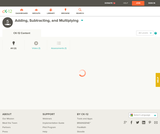Conditional Remix & Share Permitted
CC BY-NC-SA
Rating
0.0 stars

This short video and interactive assessment activity is designed to teach fifth graders about adding, subtracting, and multiplying.

Subject:
Mathematics
Numbers and Operations
Material Type:
Assessment
Interactive
Lecture
Provider:
CK-12 Foundation
Provider Set:
CK-12 Elementary Math
Date Added:
03/06/2015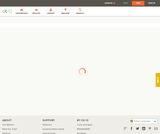Conditional Remix & Share Permitted
CC BY-NC-SA
Rating
0.0 stars

This short video and interactive assessment activity is designed to teach fifth graders about adding and subtracting.

Subject:
Mathematics
Numbers and Operations
Material Type:
Assessment
Interactive
Lecture
Provider:
CK-12 Foundation
Provider Set:
CK-12 Elementary Math
Date Added:
03/06/2015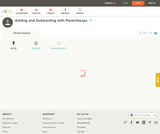Conditional Remix & Share Permitted
CC BY-NC-SA
Rating
0.0 stars

This short video and interactive assessment activity is designed to teach fifth graders about adding and subtracting with parentheses.

Subject:
Mathematics
Numbers and Operations
Material Type:
Assessment
Interactive
Lecture
Provider:
CK-12 Foundation
Provider Set:
CK-12 Elementary Math
Date Added:
03/06/2015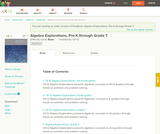Unrestricted Use
CC BY
Rating
0.0 stars

CK-12 Algebra Explorations is a hands-on series of activities that guides students from Pre-K to Grade 7 through algebraic concepts.

Subject:
Algebra
Functions
Material Type:
Activity/Lab
Lesson Plan
Textbook
Provider:
CK-12 Foundation
Provider Set:
CK-12 FlexBook
Author:
Mary Cavanagh, Carol Findell, Carole Greenes
Date Added:
10/04/2011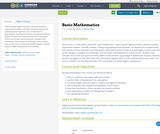Unrestricted Use
CC BY
Rating
0.0 stars

Topics include signed numbers, decimal numbers, exponential notation, scientific notation, solving and graphing linear equations, an introduction to polynomials, and systems of linear equations and their graphs. Geometrical topics include lines and angles, closed curves and convex polygons, triangles and similarities, and symmetry and proportion in nature and art. All course content by John McColgan. Content added to OER Commons by Victoria Vidal.

Subject:
Mathematics
Numbers and Operations
Material Type:
Syllabus
Author:
Victoria Vidal
Date Added:
10/10/2019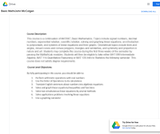Unrestricted Use
CC BY
Rating
0.0 stars

This course is a continuation of MAT087, Basic Mathematics. Topics include signed numbers, decimal numbers, exponential notation, scientific notation, solving and graphing linear equations, an introduction to polynomials, and systems of linear equations and their graphs. Geometrical topics include lines and angles, closed curves and convex polygons, triangles and similarities, and symmetry and proportion in nature and art. Students may complete this course during the first three weeks of the semester by passing the MyMathLab modules. Students will then be eligible to take either MAT 099 Intermediate Algebra, MAT 114-Quantitative Reasoning or MAT 120-Intro to Statistics the following semester. This course does not satisfy degree requirements.

Subject:
Mathematics
Material Type:
Full Course
Provider:
Roxbury Community College
Author:
John McColgan
Date Added:
05/15/2019Conditional Remix & Share Permitted
CC BY-NC
Rating
5.0 stars

This unit is an EQuIP Exemplar for adult education (http://achieve.org/equip). Students will connect their prior, real-world knowledge to the concept of order in mathematics. They will go through a discovery process with content that will build a deep, conceptual understanding of the properties of operations to explain why we perform operations in a certain order when we see just the naked numbers.

Material Type:
Lesson Plan
Unit of Study
Provider:
EQuIP Exemplars
Author:
Connie Rivera
Date Added:
11/26/2014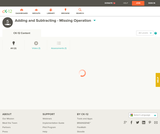Conditional Remix & Share Permitted
CC BY-NC-SA
Rating
0.0 stars

This short video and interactive assessment activity is designed to teach fifth graders about adding and subtracting - missing operation.

Subject:
Mathematics
Numbers and Operations
Material Type:
Assessment
Interactive
Lecture
Provider:
CK-12 Foundation
Provider Set:
CK-12 Elementary Math
Date Added:
03/06/2015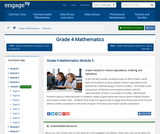Conditional Remix & Share Permitted
CC BY-NC-SA
Rating
0.0 stars

In this 40-day module, students build on their Grade 3 work with unit fractions as they explore fraction equivalence and extend this understanding to mixed numbers.  This leads to the comparison of fractions and mixed numbers and the representation of both in a variety of models.  Benchmark fractions play an important part in students ability to generalize and reason about relative fraction and mixed number sizes.  Students then have the opportunity to apply what they know to be true for whole number operations to the new concepts of fraction and mixed number operations.

Subject:
Ratios and Proportions
Material Type:
Module
Provider:
New York State Education Department
Provider Set:
EngageNY
Date Added:
01/17/2014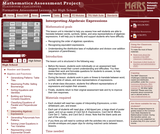Only Sharing Permitted
CC BY-NC-ND
Rating
0.0 stars

This lesson unit is intended to help teachers assess how well students are able to translate between words, symbols, tables, and area representations of algebraic expressions. It will help teachers to identify and support students who have difficulty in: recognizing the order of algebraic operations; recognizing equivalent expressions; and understanding the distributive laws of multiplication and division over addition (expansion of parentheses).

Subject:
Algebra
Material Type:
Assessment
Lesson Plan
Provider:
Shell Center for Mathematical Education
Provider Set:
Mathematics Assessment Project (MAP)
Date Added:
04/26/2013Only Sharing Permitted
CC BY-NC-ND
Rating
4.0 stars

This lesson unit is intended to help you assess how well students are able to: Perform arithmetic operations, including those involving whole-number exponents, recognizing and applying the conventional order of operations; Write and evaluate numerical expressions from diagrammatic representations and be able to identify equivalent expressions; apply the distributive and commutative properties appropriately; and use the method for finding areas of compound rectangles.

Subject:
Geometry
Material Type:
Assessment
Lesson Plan
Provider:
Shell Center for Mathematical Education
Provider Set:
Mathematics Assessment Project (MAP)
Date Added:
04/26/2013Conditional Remix & Share Permitted
CC BY-NC
Rating
0.0 stars

Subject:
Mathematics
Material Type:
Full Course
Provider:
Pearson
Date Added:
10/06/2016Rating
0.0 stars

Expressions

Type of Unit: Concept

Prior Knowledge

Students should be able to:

Write and evaluate simple expressions that record calculations with numbers.
Use parentheses, brackets, or braces in numerical expressions and evaluate expressions with these symbols.
Interpret numerical expressions without evaluating them.

Lesson Flow

Students learn to write and evaluate numerical expressions involving the four basic arithmetic operations and whole-number exponents. In specific contexts, they create and interpret numerical expressions and evaluate them. Then students move on to algebraic expressions, in which letters stand for numbers. In specific contexts, students simplify algebraic expressions and evaluate them for given values of the variables. Students learn about and use the vocabulary of algebraic expressions. Then they identify equivalent expressions and apply properties of operations, such as the distributive property, to generate equivalent expressions. Finally, students use geometric models to explore greatest common factors and least common multiples.

Subject:
Mathematics
Algebra
Material Type:
Unit of Study
Provider:
PearsonConditional Remix & Share Permitted
CC BY-NC
Rating
0.0 stars

Students express the lengths of trains as algebraic expressions and then substitute numbers for letters to find the actual lengths of the trains.Key ConceptsAn algebraic expression can be written to represent a problem situation. More than one algebraic expression may represent the same problem situation. These algebraic expressions have the same value and are equivalent.To evaluate an algebraic expression, a specific value for each variable is substituted in the expression, and then all the calculations are completed using the order of operations to get a single value.Goals and Learning ObjectivesEvaluate expressions for the given values of the variables.

Subject:
Algebra
Material Type:
Lesson Plan
Provider:
Pearson
Date Added:
09/21/2015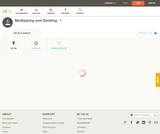Conditional Remix & Share Permitted
CC BY-NC-SA
Rating
0.0 stars

This short video and interactive assessment activity is designed to teach fifth graders about multiplying and dividing.

Subject:
Mathematics
Numbers and Operations
Material Type:
Assessment
Interactive
Lecture
Provider:
CK-12 Foundation
Provider Set:
CK-12 Elementary Math
Date Added:
03/06/2015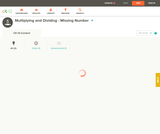Conditional Remix & Share Permitted
CC BY-NC-SA
Rating
0.0 stars

This short video and interactive assessment activity is designed to teach fifth graders about multiplying and dividing - missing number.

Subject:
Mathematics
Numbers and Operations
Material Type:
Assessment
Interactive
Lecture
Provider:
CK-12 Foundation
Provider Set:
CK-12 Elementary Math
Date Added:
03/06/2015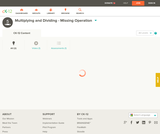Conditional Remix & Share Permitted
CC BY-NC-SA
Rating
0.0 stars

This short video and interactive assessment activity is designed to teach fifth graders about multiplying and dividing - missing operation.

Subject:
Mathematics
Numbers and Operations
Material Type:
Assessment
Interactive
Lecture
Provider:
CK-12 Foundation
Provider Set:
CK-12 Elementary Math
Date Added:
03/06/2015
Remix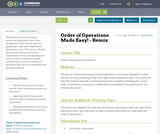Unrestricted Use
CC BY
Rating
0.0 stars

This lesson is about evaluating numerical expressions, and it was designed for adult learners who are preparing to take their High School Equivalency tests. This course will help the students evaluate numerical expressions correctly by following the correct order of operations, which includes the four basic arithmetical operations and the use of exponents and grouping symbols.

Subject:
Mathematics
Material Type:
Lesson
Author:
Susan Jones
Date Added:
08/08/2019